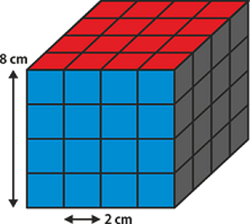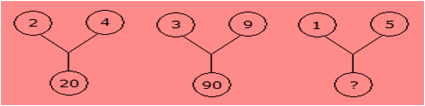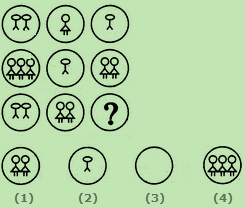# SOF - IMO PDF Sample Papers for Class 8

Class 8 sample paper & practice questions for International Mathematics Olympiad (IMO) level 1 are given below. Syllabus for level 1 is also mentioned for these exams. You can refer these sample paper & quiz for preparing for the exam.#### Resources:

##### Sample Questions from Olympiad Success:
 Q.1 Q.2 Q.3 Q.4 Q.5 Q.6 Q.7 Q.8 Q.9 Q.10
 Q.1 A solid cube of each side 8 cm, has been painted red, blue and black on pairs of opposite faces. It is then cut into cubical blocks of each side 2 cm. How many cubes have only two faces painted?a) 24 b) 2 c) 22 d) 12
 Q.2 Which one of the following number is a perfect square? a) 622 b) 393 c) 5778 d) 625
 Q.3 Which of the following is not the property of a rectangle? a) a rectangle is a parallelogram in which one of  the angles is a right angle b) diagonals of a rectangle are equal c) opposite sides of a rectangle are equal d) diagonals of a rectangle bisect each other at right angle
 Q.4 Find the missing character from among the given alternatives:a) 75 b) 20 c) 25 d) 26
 Q.5 Expansion of -8(3a + 5b) yields: a) -24a - 40b b) -22a + 80b c) 20a - 30b d) -24a - 41b
 Q.6 Select a suitable figure from the four alternatives that would complete the figure matrix.a) 1 b) 2 c) 3 d) 4
 Q.7 In equation 9x - 12k = 0. For x = 4, value of k is: a) 6 b) 5 c) 3 d) 8
 Q.8 In a two-digit number, the unit's digit is 2 more than that of the ten's digit. The sum of the digits is 27 less than the number. Find the product of the digits of the number. a) 8 b) 15 c) 24 d) 35
 Q.9 How many non-square numbers lie between 112 and 122? a) 9 b) 10 c) 8 d) 11
 Q.10 For a fixed base, if the exponent decreases by 1, the number becomes: a) 1/10th of the previous number. b) 10 times of the previous number. c) Hundredth of the previous number. d) Hundredth times of the previous number.Sample PDF of SOF - International Mathematics Olympiad (IMO) PDF Sample Papers for Class 8:

 Q.1 )a Q.2 )d Q.3 )d Q.4 )d Q.5 )a Q.6 )c Q.7 )c Q.8 )b Q.9 )c Q.10 )a

Q.1 : a | Q.2 : d | Q.3 : d | Q.4 : d | Q.5 : a | Q.6 : c | Q.7 : c | Q.8 : b | Q.9 : c | Q.10 : a